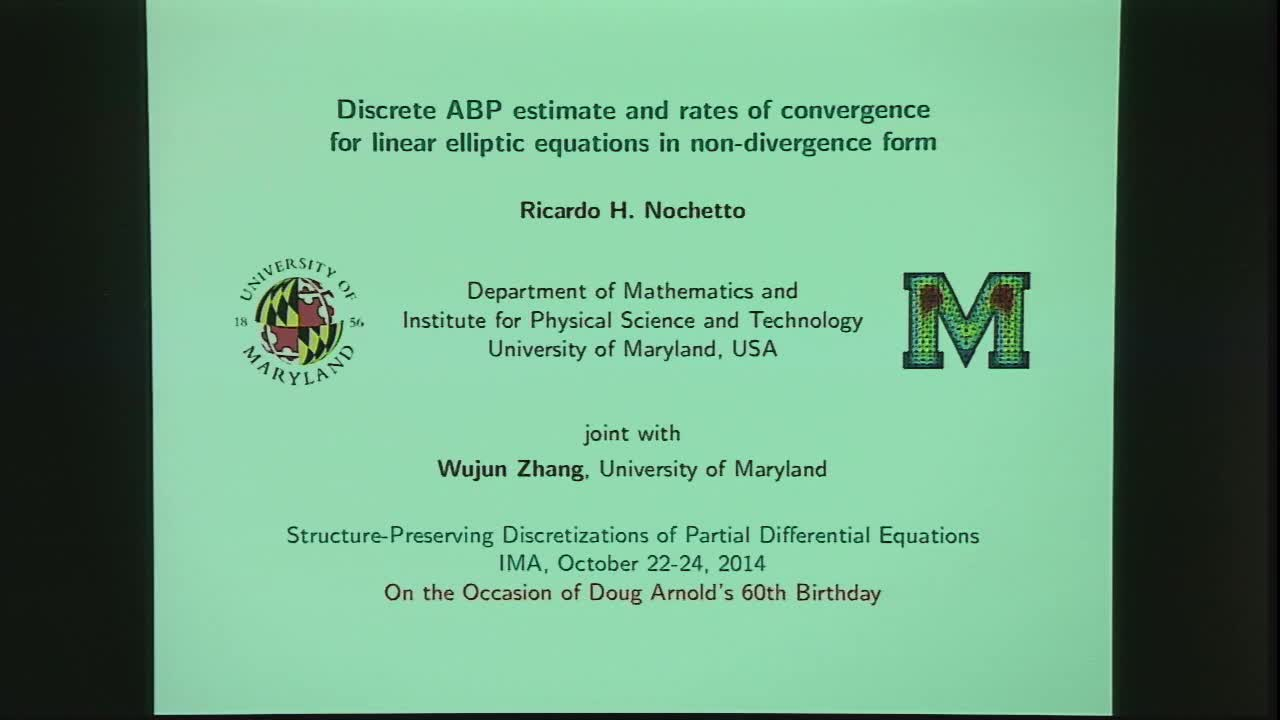## Discrete ABP Estimate and Rates of Convergence for Linear Elliptic PDEs in Non-Divergence Form

October 22, 2014
IMA
Presenters: Ricardo Nochetto

Watch Video

#### Abstract

We design a two-scale finite element method (FEM) for linear elliptic PDEs in non-divergence form. Besides the meshsize, a second larger scale is dictated by an integro-differential approximation of the PDE. We show that the FEM satisfies the discrete maximum principle (DMP) provided that the mesh is weakly acute. Combining the DMP and weak operator consistency of the FEM, we establish convergence of the numerical solution to the viscosity solution of the PDE.

We develop a discrete Alexandroff-Bakelman-Pucci (ABP) estimate which is suitable for finite element analysis. Its proof relies on a geometric interpretation of the Alexandroff estimate and control of the measure of the sub-differential of piecewise linear functions in terms of jumps, and thus of the discrete PDE. The discrete ABP estimate leads to optimal rates of convergence for our finite element method under natural regularity assumptions on the solution and coefficient matrix.

This is joint work with W. Zhang.